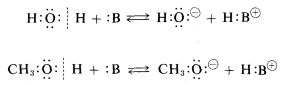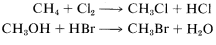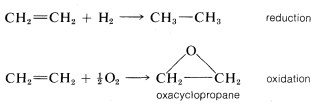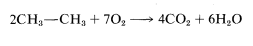# 2.3: Classification by Functional Groups

$$\newcommand{\vecs}{\overset { \rightharpoonup} {\mathbf{#1}} }$$ $$\newcommand{\vecd}{\overset{-\!-\!\rightharpoonup}{\vphantom{a}\smash {#1}}}$$$$\newcommand{\id}{\mathrm{id}}$$ $$\newcommand{\Span}{\mathrm{span}}$$ $$\newcommand{\kernel}{\mathrm{null}\,}$$ $$\newcommand{\range}{\mathrm{range}\,}$$ $$\newcommand{\RealPart}{\mathrm{Re}}$$ $$\newcommand{\ImaginaryPart}{\mathrm{Im}}$$ $$\newcommand{\Argument}{\mathrm{Arg}}$$ $$\newcommand{\norm}{\| #1 \|}$$ $$\newcommand{\inner}{\langle #1, #2 \rangle}$$ $$\newcommand{\Span}{\mathrm{span}}$$ $$\newcommand{\id}{\mathrm{id}}$$ $$\newcommand{\Span}{\mathrm{span}}$$ $$\newcommand{\kernel}{\mathrm{null}\,}$$ $$\newcommand{\range}{\mathrm{range}\,}$$ $$\newcommand{\RealPart}{\mathrm{Re}}$$ $$\newcommand{\ImaginaryPart}{\mathrm{Im}}$$ $$\newcommand{\Argument}{\mathrm{Arg}}$$ $$\newcommand{\norm}{\| #1 \|}$$ $$\newcommand{\inner}{\langle #1, #2 \rangle}$$ $$\newcommand{\Span}{\mathrm{span}}$$$$\newcommand{\AA}{\unicode[.8,0]{x212B}}$$

There are a number of recurring types of structural features in organic compounds that commonly are known as functional groups. In fact, a traditional approach to the subject of organic chemistry involves the classification of compounds according to their functional groups. Thus the structural features $$C = C$$, $$C \equiv C$$, $$C = O$$, $$OH$$, $$NH_2$$, and $$C \equiv N$$ are the functional groups of alkenes, alkynes, carbonyl compounds, alcohols, amines, and nitriles, respectively. It will be helpful to look at the structural features of some of the major types of organic compounds even though the details of their chemistry will not be discussed until later chapters. Examples of the structures arranged in accord with their functional groups are given in Table 2-2. The examples chosen are representative of compounds containing carbon and hydrogen (hydrocarbons) as well as compounds containing halogens, oxygen, nitrogen, and sulfur. We do not expect you to memorize this table. In time you will become familiar with all of the types of structures in it.

In Table 2-2 we generally have used systematic names as first-choice names because these names emphasize the relationships between the compounds and ease the burden fo the beginning student in having to remember many special names. We have little hope that systematic names such as methanal, 2-propanone, and ethanoic acid soon will replace the commonly used nonsystematic names formaldehyde, acetone, and acetic acid. But there is no question that every organic chemist knows what compounds the names methanal, 2-propanone, and ethanoic acid represent, so the beginner can communicate with these names and later become familiar with and use the special names. We will have more to say on this subject in Chapter 3.## How Are Functional-Group Classifications Useful?

One of the main reasons for classifying compounds by their functional groups is that it also classifies their chemical behavior. By this we mean that the reactions of compounds and, to some extent, their physical properties are influenced profoundly by the nature of the functional groups present. Indeed, many organic reactions involve transformations of the functional group that do not affect the rest of the molecule. For instance, alcohols, $$R-OH$$, can be transformed into a number of other compounds, such as organic halides, $$R-Cl$$ or $$R-Br$$; ethers, $$R-O-R$$; and amines, $$R-NH_2$$ without changing the structure of the hydrocarbon group $$R$$. Furthermore, any compound possessing a particular functional group may be expected to exhibit reactions characteristic of that group and, to some extent at least, of inorganic compounds with similar functional groups.

A good example of the use of the functional-group concept is for acid-base properties. Alcohols, $$ROH$$, are structurally related to water, $$HOH$$, in that both possess a hydroxyl function. We may then expect the chemistry of alcohols to be similar to that of water. In fact, both are weak acids because the $$OH$$ group has a reactive proton that it can donate to a sufficiently strongly basic substance, written as $$:B$$ here:Water and alcohols both are weak bases because the oxygens of their $$OH$$ groups have unshared electron pairs to use in bonding with a proton donated by an acid, $$HA$$:We can carry the analogy further to include carboxylic acids, $$RCO_2H$$, which also have a hydroxyl function. They also should possess acidic and basic properties. They do have these properties and they are, in fact, stronger acids than either water or alcohols and form salts with bases:Amines, $$RNH_2$$, are structurally related to ammonia, $$NH_3$$, and we therefore may predict that they will have similar properties. A property of ammonia that you probably will have encountered earlier is that it acts as a base and forms salts with acids. Amines behave likewise:It is with logic of this kind - inferring chemical behavior from structural analogies - that much of organic chemistry can be understood. There are other logical classification schemes, however, and one of these depends more on types of reactions than on functional groups.

## Classifications by Reaction Types

The rationale of classification by reaction types is that different functional groups may show the same kinds of reactions. Thus, as we have just seen, alcohols, carboxylic acids, and amines all can accept a proton from a suitably strong acid. Fortunately, there are very few different types of organic reactions - at least as far as the overall result that they produce. The most important are acid-base, substitution, addition, elimination, and rearrangement reactions. Some examples of these are given below, and you should understand that these are descriptive of the overall chemical change and nothing is implied as to how or why the reaction occurs (also see Section 1-1I).

Substitution of one atom or group of atoms for another:Addition, usually to a double or triple bond:Elimination, which is the reverse of addition:Rearrangement where one structure is converted to an isomeric structure:Certain reactions commonly are described as either oxidation or reduction reactions and most simply can be thought of as reactions that result in changes in the oxygen or hydrogen content of a molecule by direct or indirect reactions with oxygen or hydrogen, respectively. They frequently fall into one of the categories already mentioned. Reduction of ethene to ethane is clearly addition, as is oxidation of ethene to oxacyclopropane:Reactions that lead to substantial degradation of molecules into smaller fragments are more difficult to classify. An example is the combustion of ethane to carbon dioxide and water. All of the chemical bonds in the reactants are broken in this reaction. It seems pointless to try to classify this as anything but a complete combustion or oxidation reaction: